Smartick is an advanced online program that teaches kids math and coding in only 15 min. a day

Feb26

# Learn Your Times Tables at a Glance with a 100 Square

In today’s post we’re going to represent the results of the times tables in a “100 square”. Learning your times tables will be much easier with this visual aid!

A “100 square” is nothing more than a table of the numbers 1 to 100 arranged in rows of 10 by 10, like this: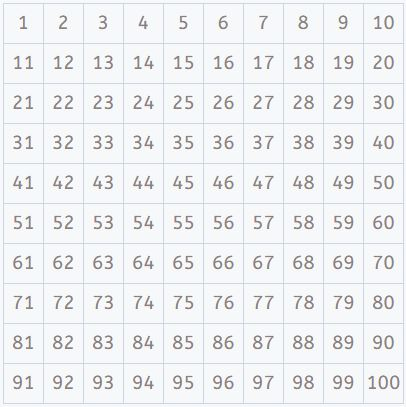We’re going to see what patterns we can create in the table if we highlight the results of the times tables. It’s something you can try yourself with this table that you can download here.

It’s an activity that makes learning your times tables easier, because it helps with understanding their behavior and construction.

As part of our Smartick sessions, we often include exercises where a table like this is shown and students can select the numbers in it, for example to select the multiples of a number: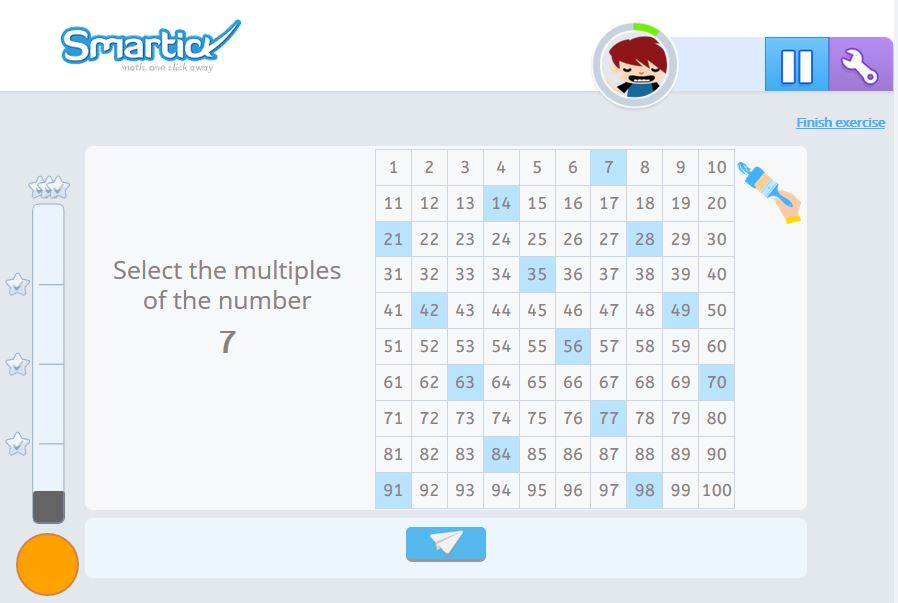#### The 2 times table

We’ll start with the 2 times table. To represent it, first we have to calculate all the multiplications in the table and write down the results:

2 x 1 = 2, 2 x 2 = 4, 2 x 3 = 6, 2 x 4 = 8, 2 x 5 = 10, 2 x 6 = 12, 2 x 7 = 14, 2 x 8 = 16, 2 x 9 = 18, 2 x 10 = 20

Then, we highlight these results in the 100 square: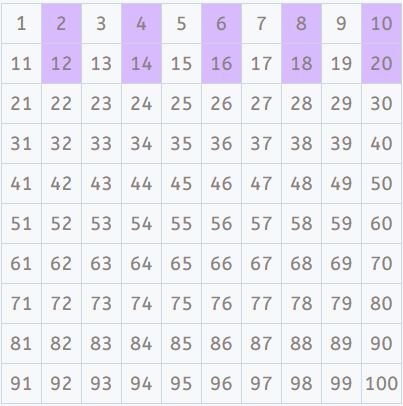As you can see, starting from the number 2 we’ve highlighted one number and left the next blank. We’ve highlighted the numbers in “jumps of 2”.

So, without having to calculate, we can mark all the results of the “extended” 2 times table, until we reach 100,  just by observing the pattern.

We’ve found the pattern for the 2 times table! It looks like this: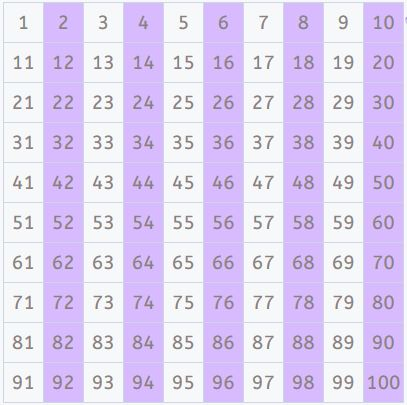You might have noticed that the 2 times table makes a very simple pattern. It’s really easy to see all the pairs highlighted in a 100 square.

This makes it easy to memorize the results and learn one of your times tables!

#### The 3 times table

We’ll continue with the 3 times table. Again, to represent it, calculate all the multiplications in the table and write down the results:

3 x 1 = 3, 3 x 2 = 6, 3 x 3 = 9, 3 x 4 = 12, 3 x 5 = 15, 3 x 6 = 18, 3 x 7 = 21, 3 x 8 = 24, 3 x 9 = 27, 3 x 10 = 30

As before, highlight these results in the 100 square: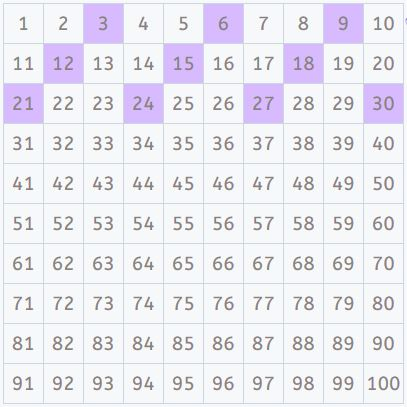Once again, if we follow the pattern that’s being created, we can highlight all the results of the “extended” 3 times table until we reach 100.

We’ve found the pattern for the 3 times table! Here’s what it looks like: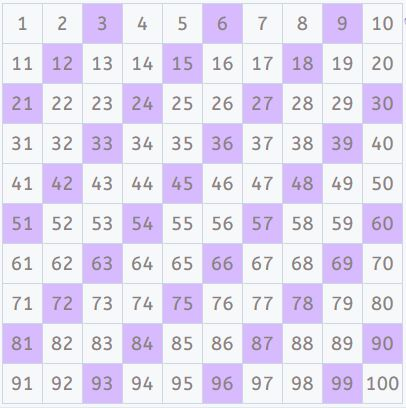Lovely, isn’t it? Just like before, you can easily memorize the results and learn the 3 times table.

#### The 4 times table

Now it’s your turn to represent the 4 times table. You can do it with the 100 square that you can download here.

If you find it too hard, do the 5 times table first and then give it another try, it’ll be much easier!

Did you like the idea of representing the times tables on a 100 square? Do you think it’s useful for memorizing, understanding and learning times tables?

If you liked this post, share it so others can enjoy these visualization exercises too!

With Smartick, you can learn more about times tables and other mathematical concepts, and work on exercises that adapt to each student in real time. Try it FREE!